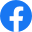(843) 839-0702
0 Items
(843) 839-0702
``` ```
``` window.NREUM||(NREUM={});NREUM.info = {"beacon":"bam.nr-data.net","errorBeacon":"bam.nr-data.net","licenseKey":"5515df9122","applicationID":"3984636","transactionName":"MgQGYEFTDUtQUU1dWQtOKWJwHTddXEJVVUIAIgtaR0AMVF1XSxtyChYKWFxTB3BFX1U=","queueTime":0,"applicationTime":24,"agent":"","atts":"HkMFFglJQVZDHE1GXxUoABYJEFIJdXEABgQhU1wFdwNWDHAQRBgUEENeTxFHEF1DXFhZU0dbRl5cV01TRFxSUVolBhZVUFcFTV1eQFVRDA8DGlxABBodEFZGUQQPDU5SRgpXXxADFngACANcUV0RGEVdGXpTDAYMVlxAQ1dXEmpbQxEJRHdSQAxUWFxYFhpHCBR1V1YRXUJBGw4UU1VKBQAKTQoEBhcNAkdNRkZWQxZdQkZwUBRfQz8GUQJXXANWXBlXU1VWGQcDVwkcUwoCAEgDUgUHUVcJCVMIUQc4QxlJ"}(window.NREUM||(NREUM={})).loader_config={xpid:"Ug8OUVBVGwAJXFVUBAc=",licenseKey:"5515df9122",applicationID:"3984636"};window.NREUM||(NREUM={}),__nr_require=function(t,n,e){function r(e){if(!n[e]){var o=n[e]={exports:{}};t[e].call(o.exports,function(n){var o=t[e][n];return r(o||n)},o,o.exports)}return n[e].exports}if("function"==typeof __nr_require)return __nr_require;for(var o=0;o<e.length;o++)r(e[o]);return r}({1:[function(t,n,e){function r(t){try{s.console&#038;&#038;console.log(t)}catch(n){}}var o,i=t("ee"),a=t(20),s={};try{o=localStorage.getItem("__nr_flags").split(","),console&#038;&#038;"function"==typeof console.log&#038;&#038;(s.console=!0,o.indexOf("dev")!==-1&#038;&#038;(s.dev=!0),o.indexOf("nr_dev")!==-1&#038;&#038;(s.nrDev=!0))}catch(c){}s.nrDev&#038;&#038;i.on("internal-error",function(t){r(t.stack)}),s.dev&#038;&#038;i.on("fn-err",function(t,n,e){r(e.stack)}),s.dev&#038;&#038;(r("NR AGENT IN DEVELOPMENT MODE"),r("flags: "+a(s,function(t,n){return t}).join(", ")))},{}],2:[function(t,n,e){function r(t,n,e,r,s){try{p?p-=1:o(s||new UncaughtException(t,n,e),!0)}catch(f){try{i("ierr",[f,c.now(),!0])}catch(d){}}return"function"==typeof u&#038;&#038;u.apply(this,a(arguments))}function UncaughtException(t,n,e){this.message=t||"Uncaught error with no additional information",this.sourceURL=n,this.line=e}function o(t,n){var e=n?null:c.now();i("err",[t,e])}var i=t("handle"),a=t(21),s=t("ee"),c=t("loader"),f=t("gos"),u=window.onerror,d=!1,l="nr@seenError",p=0;c.features.err=!0,t(1),window.onerror=r;try{throw new Error}catch(h){"stack"in h&#038;&#038;(t(9),t(8),"addEventListener"in window&#038;&#038;t(5),c.xhrWrappable&#038;&#038;t(10),d=!0)}s.on("fn-start",function(t,n,e){d&#038;&#038;(p+=1)}),s.on("fn-err",function(t,n,e){d&#038;&#038;!e[l]&#038;&#038;(f(e,l,function(){return!0}),this.thrown=!0,o(e))}),s.on("fn-end",function(){d&#038;&#038;!this.thrown&#038;&#038;p>0&&(p-=1)}),s.on("internal-error",function(t){i("ierr",[t,c.now(),!0])})},{}],3:[function(t,n,e){t("loader").features.ins=!0},{}],4:[function(t,n,e){function r(t){}if(window.performance&&window.performance.timing&&window.performance.getEntriesByType){var o=t("ee"),i=t("handle"),a=t(9),s=t(8),c="learResourceTimings",f="addEventListener",u="resourcetimingbufferfull",d="bstResource",l="resource",p="-start",h="-end",m="fn"+p,w="fn"+h,v="bstTimer",g="pushState",y=t("loader");y.features.stn=!0,t(7),"addEventListener"in window&&t(5);var x=NREUM.o.EV;o.on(m,function(t,n){var e=t;e instanceof x&&(this.bstStart=y.now())}),o.on(w,function(t,n){var e=t;e instanceof x&&i("bst",[e,n,this.bstStart,y.now()])}),a.on(m,function(t,n,e){this.bstStart=y.now(),this.bstType=e}),a.on(w,function(t,n){i(v,[n,this.bstStart,y.now(),this.bstType])}),s.on(m,function(){this.bstStart=y.now()}),s.on(w,function(t,n){i(v,[n,this.bstStart,y.now(),"requestAnimationFrame"])}),o.on(g+p,function(t){this.time=y.now(),this.startPath=location.pathname+location.hash}),o.on(g+h,function(t){i("bstHist",[location.pathname+location.hash,this.startPath,this.time])}),f in window.performance&&(window.performance["c"+c]?window.performance[f](u,function(t){i(d,[window.performance.getEntriesByType(l)]),window.performance["c"+c]()},!1):window.performance[f]("webkit"+u,function(t){i(d,[window.performance.getEntriesByType(l)]),window.performance["webkitC"+c]()},!1)),document[f]("scroll",r,{passive:!0}),document[f]("keypress",r,!1),document[f]("click",r,!1)}},{}],5:[function(t,n,e){function r(t){for(var n=t;n&&!n.hasOwnProperty(u);)n=Object.getPrototypeOf(n);n&&o(n)}function o(t){s.inPlace(t,[u,d],"-",i)}function i(t,n){return t}var a=t("ee").get("events"),s=t("wrap-function")(a,!0),c=t("gos"),f=XMLHttpRequest,u="addEventListener",d="removeEventListener";n.exports=a,"getPrototypeOf"in Object?(r(document),r(window),r(f.prototype)):f.prototype.hasOwnProperty(u)&&(o(window),o(f.prototype)),a.on(u+"-start",function(t,n){var e=t,r=c(e,"nr@wrapped",function(){function t(){if("function"==typeof e.handleEvent)return e.handleEvent.apply(e,arguments)}var n={object:t,"function":e}[typeof e];return n?s(n,"fn-",null,n.name||"anonymous"):e});this.wrapped=t=r}),a.on(d+"-start",function(t){t=this.wrapped||t})},{}],6:[function(t,n,e){function r(t,n,e){var r=t[n];"function"==typeof r&&(t[n]=function(){var t=i(arguments),n={};o.emit(e+"before-start",[t],n);var a;n[m]&&n[m].dt&&(a=n[m].dt);var s=r.apply(this,t);return o.emit(e+"start",[t,a],s),s.then(function(t){return o.emit(e+"end",[null,t],s),t},function(t){throw o.emit(e+"end",[t],s),t})})}var o=t("ee").get("fetch"),i=t(21),a=t(20);n.exports=o;var s=window,c="fetch-",f=c+"body-",u=["arrayBuffer","blob","json","text","formData"],d=s.Request,l=s.Response,p=s.fetch,h="prototype",m="nr@context";d&&l&&p&&(a(u,function(t,n){r(d[h],n,f),r(l[h],n,f)}),r(s,"fetch",c),o.on(c+"end",function(t,n){var e=this;if(n){var r=n.headers.get("content-length");null!==r&&(e.rxSize=r),o.emit(c+"done",[null,n],e)}else o.emit(c+"done",[t],e)}))},{}],7:[function(t,n,e){var r=t("ee").get("history"),o=t("wrap-function")(r);n.exports=r;var i=window.history&&window.history.constructor&&window.history.constructor.prototype,a=window.history;i&&i.pushState&&i.replaceState&&(a=i),o.inPlace(a,["pushState","replaceState"],"-")},{}],8:[function(t,n,e){var r=t("ee").get("raf"),o=t("wrap-function")(r),i="equestAnimationFrame";n.exports=r,o.inPlace(window,["r"+i,"mozR"+i,"webkitR"+i,"msR"+i],"raf-"),r.on("raf-start",function(t){t=o(t,"fn-")})},{}],9:[function(t,n,e){function r(t,n,e){t=a(t,"fn-",null,e)}function o(t,n,e){this.method=e,this.timerDuration=isNaN(t)?0:+t,t=a(t,"fn-",this,e)}var i=t("ee").get("timer"),a=t("wrap-function")(i),s="setTimeout",c="setInterval",f="clearTimeout",u="-start",d="-";n.exports=i,a.inPlace(window,[s,"setImmediate"],s+d),a.inPlace(window,[c],c+d),a.inPlace(window,[f,"clearImmediate"],f+d),i.on(c+u,r),i.on(s+u,o)},{}],10:[function(t,n,e){function r(t,n){d.inPlace(n,["onreadystatechange"],"fn-",s)}function o(){var t=this,n=u.context(t);t.readyState>3&&!n.resolved&&(n.resolved=!0,u.emit("xhr-resolved",[],t)),d.inPlace(t,g,"fn-",s)}function i(t){y.push(t),h&&(b?b.then(a):w?w(a):(E=-E,R.data=E))}function a(){for(var t=0;t<y.length;t++)r([],y[t]);y.length&#038;&#038;(y=[])}function s(t,n){return n}function c(t,n){for(var e in t)n[e]=t[e];return n}t(5);var f=t("ee"),u=f.get("xhr"),d=t("wrap-function")(u),l=NREUM.o,p=l.XHR,h=l.MO,m=l.PR,w=l.SI,v="readystatechange",g=["onload","onerror","onabort","onloadstart","onloadend","onprogress","ontimeout"],y=[];n.exports=u;var x=window.XMLHttpRequest=function(t){var n=new p(t);try{u.emit("new-xhr",[n],n),n.addEventListener(v,o,!1)}catch(e){try{u.emit("internal-error",[e])}catch(r){}}return n};if(c(p,x),x.prototype=p.prototype,d.inPlace(x.prototype,["open","send"],"-xhr-",s),u.on("send-xhr-start",function(t,n){r(t,n),i(n)}),u.on("open-xhr-start",r),h){var b=m&#038;&#038;m.resolve();if(!w&#038;&#038;!m){var E=1,R=document.createTextNode(E);new h(a).observe(R,{characterData:!0})}}else f.on("fn-end",function(t){t&#038;&#038;t.type===v||a()})},{}],11:[function(t,n,e){function r(){var t=window.NREUM;if(!t.loader_config)return null;var n=(t.loader_config.accountID||"").toString()||null,e=(t.loader_config.agentID||"").toString()||null,r=(t.loader_config.trustKey||"").toString()||null;if(!n||!e)return null;var a=i.generateCatId(),s=i.generateCatId(),c=Date.now(),f=o(a,s,c,n,e,r);return{header:f,guid:a,traceId:s,timestamp:c}}function o(t,n,e,r,o,i){var a="btoa"in window&#038;&#038;"function"==typeof window.btoa;if(!a)return null;var s={v:[0,1],d:{ty:"Browser",ac:r,ap:o,id:t,tr:n,ti:e}};return i&#038;&#038;r!==i&#038;&#038;(s.d.tk=i),btoa(JSON.stringify(s))}var i=t(18);n.exports={generateTracePayload:r,generateTraceHeader:o}},{}],12:[function(t,n,e){function r(t){var n=this.params,e=this.metrics;if(!this.ended){this.ended=!0;for(var r=0;r<p;r++)t.removeEventListener(l[r],this.listener,!1);n.aborted||(e.duration=s.now()-this.startTime,this.loadCaptureCalled||4!==t.readyState?null==n.status&#038;&#038;(n.status=0):a(this,t),e.cbTime=this.cbTime,d.emit("xhr-done",[t],t),c("xhr",[n,e,this.startTime]))}}function o(t,n){var e=t.responseType;if("json"===e&#038;&#038;null!==n)return n;var r="arraybuffer"===e||"blob"===e||"json"===e?t.response:t.responseText;return w(r)}function i(t,n){var e=f(n),r=t.params;r.host=e.hostname+":"+e.port,r.pathname=e.pathname,t.sameOrigin=e.sameOrigin}function a(t,n){t.params.status=n.status;var e=o(n,t.lastSize);if(e&#038;&#038;(t.metrics.rxSize=e),t.sameOrigin){var r=n.getResponseHeader("X-NewRelic-App-Data");r&#038;&#038;(t.params.cat=r.split(", ").pop())}t.loadCaptureCalled=!0}var s=t("loader");if(s.xhrWrappable){var c=t("handle"),f=t(13),u=t(11).generateTracePayload,d=t("ee"),l=["load","error","abort","timeout"],p=l.length,h=t("id"),m=t(16),w=t(15),v=window.XMLHttpRequest;s.features.xhr=!0,t(10),t(6),d.on("new-xhr",function(t){var n=this;n.totalCbs=0,n.called=0,n.cbTime=0,n.end=r,n.ended=!1,n.xhrGuids={},n.lastSize=null,n.loadCaptureCalled=!1,t.addEventListener("load",function(e){a(n,t)},!1),m&#038;&#038;(m>34||m<10)||window.opera||t.addEventListener("progress",function(t){n.lastSize=t.loaded},!1)}),d.on("open-xhr-start",function(t){this.params={method:t},i(this,t),this.metrics={}}),d.on("open-xhr-end",function(t,n){"loader_config"in NREUM&#038;&#038;"xpid"in NREUM.loader_config&#038;&#038;this.sameOrigin&#038;&#038;n.setRequestHeader("X-NewRelic-ID",NREUM.loader_config.xpid);var e=!1;if("init"in NREUM&#038;&#038;"distributed_tracing"in NREUM.init&#038;&#038;(e=!!NREUM.init.distributed_tracing.enabled),e&#038;&#038;this.sameOrigin){var r=u();r&#038;&#038;r.header&#038;&#038;(n.setRequestHeader("newrelic",r.header),this.dt=r)}}),d.on("send-xhr-start",function(t,n){var e=this.metrics,r=t,o=this;if(e&#038;&#038;r){var i=w(r);i&#038;&#038;(e.txSize=i)}this.startTime=s.now(),this.listener=function(t){try{"abort"!==t.type||o.loadCaptureCalled||(o.params.aborted=!0),("load"!==t.type||o.called===o.totalCbs&#038;&#038;(o.onloadCalled||"function"!=typeof n.onload))&#038;&#038;o.end(n)}catch(e){try{d.emit("internal-error",[e])}catch(r){}}};for(var a=0;a<p;a++)n.addEventListener(l[a],this.listener,!1)}),d.on("xhr-cb-time",function(t,n,e){this.cbTime+=t,n?this.onloadCalled=!0:this.called+=1,this.called!==this.totalCbs||!this.onloadCalled&#038;&#038;"function"==typeof e.onload||this.end(e)}),d.on("xhr-load-added",function(t,n){var e=""+h(t)+!!n;this.xhrGuids&#038;&#038;!this.xhrGuids[e]&#038;&#038;(this.xhrGuids[e]=!0,this.totalCbs+=1)}),d.on("xhr-load-removed",function(t,n){var e=""+h(t)+!!n;this.xhrGuids&#038;&#038;this.xhrGuids[e]&#038;&#038;(delete this.xhrGuids[e],this.totalCbs-=1)}),d.on("addEventListener-end",function(t,n){n instanceof v&#038;&#038;"load"===t&#038;&#038;d.emit("xhr-load-added",[t,t],n)}),d.on("removeEventListener-end",function(t,n){n instanceof v&#038;&#038;"load"===t&#038;&#038;d.emit("xhr-load-removed",[t,t],n)}),d.on("fn-start",function(t,n,e){n instanceof v&#038;&#038;("onload"===e&#038;&#038;(this.onload=!0),("load"===(t&#038;&#038;t.type)||this.onload)&#038;&#038;(this.xhrCbStart=s.now()))}),d.on("fn-end",function(t,n){this.xhrCbStart&#038;&#038;d.emit("xhr-cb-time",[s.now()-this.xhrCbStart,this.onload,n],n)}),d.on("fetch-before-start",function(t){var n,e=t||{};"string"==typeof t?n=t:t&#038;&#038;t.url&#038;&#038;(n=t.url),n&#038;&#038;(this.sameOrigin=f(n).sameOrigin);var r=!1;if("init"in NREUM&#038;&#038;"distributed_tracing"in NREUM.init&#038;&#038;(r=!!NREUM.init.distributed_tracing.enabled),r&#038;&#038;this.sameOrigin){var o=u();if(!o||!o.header)return;var i=o.header;if("string"==typeof t){var a={};for(var s in e)a[s]=e[s];a.headers=new Headers(e.headers||{}),a.headers.set("newrelic",i),this.dt=o,t.length>1?t=a:t.push(a)}else t&&t.headers&&(t.headers.append("newrelic",i),this.dt=o)}})}},{}],13:[function(t,n,e){n.exports=function(t){var n=document.createElement("a"),e=window.location,r={};n.href=t,r.port=n.port;var o=n.href.split("://");!r.port&&o&&(r.port=o.split("/").split("@").pop().split(":")),r.port&&"0"!==r.port||(r.port="https"===o?"443":"80"),r.hostname=n.hostname||e.hostname,r.pathname=n.pathname,r.protocol=o,"/"!==r.pathname.charAt(0)&&(r.pathname="/"+r.pathname);var i=!n.protocol||":"===n.protocol||n.protocol===e.protocol,a=n.hostname===document.domain&&n.port===e.port;return r.sameOrigin=i&&(!n.hostname||a),r}},{}],14:[function(t,n,e){function r(){}function o(t,n,e){return function(){return i(t,[f.now()].concat(s(arguments)),n?null:this,e),n?void 0:this}}var i=t("handle"),a=t(20),s=t(21),c=t("ee").get("tracer"),f=t("loader"),u=NREUM;"undefined"==typeof window.newrelic&&(newrelic=u);var d=["setPageViewName","setCustomAttribute","setErrorHandler","finished","addToTrace","inlineHit","addRelease"],l="api-",p=l+"ixn-";a(d,function(t,n){u[n]=o(l+n,!0,"api")}),u.addPageAction=o(l+"addPageAction",!0),u.setCurrentRouteName=o(l+"routeName",!0),n.exports=newrelic,u.interaction=function(){return(new r).get()};var h=r.prototype={createTracer:function(t,n){var e={},r=this,o="function"==typeof n;return i(p+"tracer",[f.now(),t,e],r),function(){if(c.emit((o?"":"no-")+"fn-start",[f.now(),r,o],e),o)try{return n.apply(this,arguments)}catch(t){throw c.emit("fn-err",[arguments,this,t],e),t}finally{c.emit("fn-end",[f.now()],e)}}}};a("actionText,setName,setAttribute,save,ignore,onEnd,getContext,end,get".split(","),function(t,n){h[n]=o(p+n)}),newrelic.noticeError=function(t,n){"string"==typeof t&&(t=new Error(t)),i("err",[t,f.now(),!1,n])}},{}],15:[function(t,n,e){n.exports=function(t){if("string"==typeof t&&t.length)return t.length;if("object"==typeof t){if("undefined"!=typeof ArrayBuffer&&t instanceof ArrayBuffer&&t.byteLength)return t.byteLength;if("undefined"!=typeof Blob&&t instanceof Blob&&t.size)return t.size;if(!("undefined"!=typeof FormData&&t instanceof FormData))try{return JSON.stringify(t).length}catch(n){return}}}},{}],16:[function(t,n,e){var r=0,o=navigator.userAgent.match(/Firefox[\/\s](\d+\.\d+)/);o&&(r=+o),n.exports=r},{}],17:[function(t,n,e){function r(t,n){var e=t.getEntries();e.forEach(function(t){"first-paint"===t.name?a("timing",["fp",Math.floor(t.startTime)]):"first-contentful-paint"===t.name&&a("timing",["fcp",Math.floor(t.startTime)])})}function o(t){if(t instanceof c&&!u){var n,e=Math.round(t.timeStamp);n=e>1e12?Date.now()-e:s.now()-e,u=!0,a("timing",["fi",e,{type:t.type,fid:n}])}}if(!("init"in NREUM&&"page_view_timing"in NREUM.init&&"enabled"in NREUM.init.page_view_timing&&NREUM.init.page_view_timing.enabled===!1)){var i,a=t("handle"),s=t("loader"),c=NREUM.o.EV;if("PerformanceObserver"in window&&"function"==typeof window.PerformanceObserver){i=new PerformanceObserver(r);try{i.observe({entryTypes:["paint"]})}catch(f){}}if("addEventListener"in document){var u=!1,d=["click","keydown","mousedown","pointerdown","touchstart"];d.forEach(function(t){document.addEventListener(t,o,!1)})}}},{}],18:[function(t,n,e){function r(){function t(){return n?15&n[e++]:16*Math.random()|0}var n=null,e=0,r=window.crypto||window.msCrypto;r&&r.getRandomValues&&(n=r.getRandomValues(new Uint8Array(31)));for(var o,i="xxxxxxxx-xxxx-4xxx-yxxx-xxxxxxxxxxxx",a="",s=0;s<i.length;s++)o=i[s],"x"===o?a+=t().toString(16):"y"===o?(o=3&#038;t()|8,a+=o.toString(16)):a+=o;return a}function o(){function t(){return n?15&#038;n[e++]:16*Math.random()|0}var n=null,e=0,r=window.crypto||window.msCrypto;r&#038;&#038;r.getRandomValues&#038;&#038;Uint8Array&#038;&#038;(n=r.getRandomValues(new Uint8Array(31)));for(var o=[],i=0;i<16;i++)o.push(t().toString(16));return o.join("")}n.exports={generateUuid:r,generateCatId:o}},{}],19:[function(t,n,e){function r(t,n){if(!o)return!1;if(t!==o)return!1;if(!n)return!0;if(!i)return!1;for(var e=i.split("."),r=n.split("."),a=0;a<r.length;a++)if(r[a]!==e[a])return!1;return!0}var o=null,i=null,a=/Version\/(\S+)\s+Safari/;if(navigator.userAgent){var s=navigator.userAgent,c=s.match(a);c&#038;&#038;s.indexOf("Chrome")===-1&#038;&#038;s.indexOf("Chromium")===-1&#038;&#038;(o="Safari",i=c)}n.exports={agent:o,version:i,match:r}},{}],20:[function(t,n,e){function r(t,n){var e=[],r="",i=0;for(r in t)o.call(t,r)&#038;&#038;(e[i]=n(r,t[r]),i+=1);return e}var o=Object.prototype.hasOwnProperty;n.exports=r},{}],21:[function(t,n,e){function r(t,n,e){n||(n=0),"undefined"==typeof e&#038;&#038;(e=t?t.length:0);for(var r=-1,o=e-n||0,i=Array(o<0?0:o);++r<o;)i[r]=t[n+r];return i}n.exports=r},{}],22:[function(t,n,e){n.exports={exists:"undefined"!=typeof window.performance&#038;&#038;window.performance.timing&#038;&#038;"undefined"!=typeof window.performance.timing.navigationStart}},{}],ee:[function(t,n,e){function r(){}function o(t){function n(t){return t&#038;&#038;t instanceof r?t:t?c(t,s,i):i()}function e(e,r,o,i){if(!l.aborted||i){t&#038;&#038;t(e,r,o);for(var a=n(o),s=m(e),c=s.length,f=0;f<c;f++)s[f].apply(a,r);var d=u[y[e]];return d&#038;&#038;d.push([x,e,r,a]),a}}function p(t,n){g[t]=m(t).concat(n)}function h(t,n){var e=g[t];if(e)for(var r=0;r<e.length;r++)e[r]===n&#038;&#038;e.splice(r,1)}function m(t){return g[t]||[]}function w(t){return d[t]=d[t]||o(e)}function v(t,n){f(t,function(t,e){n=n||"feature",y[e]=n,n in u||(u[n]=[])})}var g={},y={},x={on:p,addEventListener:p,removeEventListener:h,emit:e,get:w,listeners:m,context:n,buffer:v,abort:a,aborted:!1};return x}function i(){return new r}function a(){(u.api||u.feature)&#038;&#038;(l.aborted=!0,u=l.backlog={})}var s="nr@context",c=t("gos"),f=t(20),u={},d={},l=n.exports=o();l.backlog=u},{}],gos:[function(t,n,e){function r(t,n,e){if(o.call(t,n))return t[n];var r=e();if(Object.defineProperty&#038;&#038;Object.keys)try{return Object.defineProperty(t,n,{value:r,writable:!0,enumerable:!1}),r}catch(i){}return t[n]=r,r}var o=Object.prototype.hasOwnProperty;n.exports=r},{}],handle:[function(t,n,e){function r(t,n,e,r){o.buffer([t],r),o.emit(t,n,e)}var o=t("ee").get("handle");n.exports=r,r.ee=o},{}],id:[function(t,n,e){function r(t){var n=typeof t;return!t||"object"!==n&#038;&#038;"function"!==n?-1:t===window?0:a(t,i,function(){return o++})}var o=1,i="nr@id",a=t("gos");n.exports=r},{}],loader:[function(t,n,e){function r(){if(!E++){var t=b.info=NREUM.info,n=p.getElementsByTagName("script");if(setTimeout(u.abort,3e4),!(t&#038;&#038;t.licenseKey&#038;&#038;t.applicationID&#038;&#038;n))return u.abort();f(y,function(n,e){t[n]||(t[n]=e)}),c("mark",["onload",a()+b.offset],null,"api");var e=p.createElement("script");e.src="https://"+t.agent,n.parentNode.insertBefore(e,n)}}function o(){"complete"===p.readyState&#038;&#038;i()}function i(){c("mark",["domContent",a()+b.offset],null,"api")}function a(){return R.exists&#038;&#038;performance.now?Math.round(performance.now()):(s=Math.max((new Date).getTime(),s))-b.offset}var s=(new Date).getTime(),c=t("handle"),f=t(20),u=t("ee"),d=t(19),l=window,p=l.document,h="addEventListener",m="attachEvent",w=l.XMLHttpRequest,v=w&#038;&#038;w.prototype;NREUM.o={ST:setTimeout,SI:l.setImmediate,CT:clearTimeout,XHR:w,REQ:l.Request,EV:l.Event,PR:l.Promise,MO:l.MutationObserver};var g=""+location,y={beacon:"bam.nr-data.net",errorBeacon:"bam.nr-data.net",agent:"js-agent.newrelic.com/nr-1153.min.js"},x=w&#038;&#038;v&#038;&#038;v[h]&#038;&#038;!/CriOS/.test(navigator.userAgent),b=n.exports={offset:s,now:a,origin:g,features:{},xhrWrappable:x,userAgent:d};t(14),t(17),p[h]?(p[h]("DOMContentLoaded",i,!1),l[h]("load",r,!1)):(p[m]("onreadystatechange",o),l[m]("onload",r)),c("mark",["firstbyte",s],null,"api");var E=0,R=t(22)},{}],"wrap-function":[function(t,n,e){function r(t){return!(t&#038;&#038;t instanceof Function&#038;&#038;t.apply&#038;&#038;!t[a])}var o=t("ee"),i=t(21),a="nr@original",s=Object.prototype.hasOwnProperty,c=!1;n.exports=function(t,n){function e(t,n,e,o){function nrWrapper(){var r,a,s,c;try{a=this,r=i(arguments),s="function"==typeof e?e(r,a):e||{}}catch(f){l([f,"",[r,a,o],s])}u(n+"start",[r,a,o],s);try{return c=t.apply(a,r)}catch(d){throw u(n+"err",[r,a,d],s),d}finally{u(n+"end",[r,a,c],s)}}return r(t)?t:(n||(n=""),nrWrapper[a]=t,d(t,nrWrapper),nrWrapper)}function f(t,n,o,i){o||(o="");var a,s,c,f="-"===o.charAt(0);for(c=0;c<n.length;c++)s=n[c],a=t[s],r(a)||(t[s]=e(a,f?s+o:o,i,s))}function u(e,r,o){if(!c||n){var i=c;c=!0;try{t.emit(e,r,o,n)}catch(a){l([a,e,r,o])}c=i}}function d(t,n){if(Object.defineProperty&#038;&#038;Object.keys)try{var e=Object.keys(t);return e.forEach(function(e){Object.defineProperty(n,e,{get:function(){return t[e]},set:function(n){return t[e]=n,n}})}),n}catch(r){l([r])}for(var o in t)s.call(t,o)&#038;&#038;(n[o]=t[o]);return n}function l(n){try{t.emit("internal-error",n)}catch(e){}}return t||(t=o),e.inPlace=f,e.flag=a,e}},{}]},{},["loader",2,12,4,3]); body { margin: 0; padding: 0; }</p> <p> table, td, tr { vertical-align: top; border-collapse: collapse; }</p> <p> * { line-height: inherit; }</p> <p> a[x-apple-data-detectors=true] { color: inherit !important; text-decoration: none !important; } @media (max-width: 620px) {</p> <p> .block-grid, .col { min-width: 320px !important; max-width: 100% !important; display: block !important; }</p> <p> .block-grid { width: 100% !important; }</p> <p> .col { width: 100% !important; }</p> <p> .col>div { margin: 0 auto; }</p> <p> img.fullwidth, img.fullwidthOnMobile { max-width: 100% !important; }</p> <p> .no-stack .col { min-width: 0 !important; display: table-cell !important; }</p> <p> .no-stack.two-up .col { width: 50% !important; }</p> <p> .no-stack .col.num4 { width: 33% !important; }</p> <p> .no-stack .col.num8 { width: 66% !important; }</p> <p> .no-stack .col.num4 { width: 33% !important; }</p> <p> .no-stack .col.num3 { width: 25% !important; }</p> <p> .no-stack .col.num6 { width: 50% !important; }</p> <p> .no-stack .col.num9 { width: 75% !important; }</p> <p> .video-block { max-width: none !important; }</p> <p> .mobile_hide { min-height: 0px; max-height: 0px; max-width: 0px; display: none; overflow: hidden; font-size: 0px; }</p> <p> .desktop_hide { display: block !important; max-height: none !important; } }Hi *{{First Name}}*  My name is Joe and I am the interim Volunteer Coordinator for Neighbor to Neighbor.  I'm excited to introduce myself to you and to pick up where my colleague left off.  At Neighbor to Neighbor of South Carolina, we strive to make it easy for you to volunteer your time and to give back to those who truly need your help.  As an award winning nonprofit organization, you will have flexibility, support, supplemental insurance, and most importantly, a committed staff to assist you along your journey.  We can't wait to have you on board! Upcoming Orientation Dates Click the dates to RSVP via Facebook Tuesday, December 3rd from 10:00 am to 11:30 am.   Wednesday, December 11th from 10:00 am to 11:30 am.   Wednesday, December 18th from 10:00 am to 11:30 am.   EMAIL US NOW TO RSVP Location Information Google Maps 921 N. Kings Hwy., Myrtle Beach, SC 29577 Visit the Website Calendar of Events Blog Can't make one of the orientations on our calendar?  Don't worry!  We would love to work with you individually and get your orientation set up.  Just reach out to us directly. Ways to RSVPFacebook Events Find us on Facebook Events and Reserve a Spot! Sign Up HereContact the Office Directly We welcome your call and email with smiling faces!  Contact us through the office to set up your orientation. 843-839-0702 Email us Today! Become a volunteer with an award winning program!  See our latest award from the Secretary of State. Angel AwardOur Contact Information*{{Organization Name}}**{{Organization Address}}**{{Organization Phone}}**{{Organization Website}}* *{{Unsubscribe}}* Calendar of Orientations ```